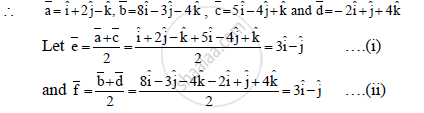HSC Arts 12th Board ExamMaharashtra State Board
Share

Books Shortlist
Your shortlist is empty

# Solution for By vector method show that the quadrilateral with vertices A (1, 2, –1), B (8, –3, –4), C (5, –4, 1), D (–2, 1, 4) is a parallelogram. - HSC Arts 12th Board Exam - Mathematics and Statistics

ConceptVectors Diagonals of a Parallelogram Bisect Each Other and Converse

#### Question

By vector method show that the quadrilateral with vertices A (1, 2, –1), B (8, –3, –4), C (5, –4, 1), D (–2, 1, 4) is a parallelogram.

#### Solution

Let bara,barb,barc and bard be the position vectors of vertices A, B, C, D respectively∴From (i) and (ii), we get

bare= barf
The mid point of the diagonals AC and BD is same
∴ The diagonals AC and BD bisect each other.
∴ The squareABCD is a Parallelogram.

Is there an error in this question or solution?

#### APPEARS IN

2015-2016 (July) (with solutions)
Question 1.2.4 | 2.00 marks

#### Video TutorialsVIEW ALL 

Solution for question: By vector method show that the quadrilateral with vertices A (1, 2, –1), B (8, –3, –4), C (5, –4, 1), D (–2, 1, 4) is a parallelogram. concept: Vectors - Diagonals of a Parallelogram Bisect Each Other and Converse. For the courses HSC Arts, HSC Science (Electronics), HSC Science (General) , HSC Science (Computer Science)
S